# probability theory

Also found in: Dictionary, Thesaurus, Wikipedia.

## probability theory

[‚präb·ə′bil·əd·ē ‚thē·ə·rē]
(mathematics)
The study of the mathematical structures and constructions used to analyze the probability of a given set of events from a family of outcomes.
McGraw-Hill Dictionary of Scientific & Technical Terms, 6E, Copyright © 2003 by The McGraw-Hill Companies, Inc.

## probability theory

See PROBABILITY.
Collins Dictionary of Sociology, 3rd ed. © HarperCollins Publishers 2000
The following article is from The Great Soviet Encyclopedia (1979). It might be outdated or ideologically biased.

## Probability Theory

a mathematical science that permits one to find, using the probabilities of some random events, the probabilities of other random events connected in some way with the first.

The assertion that a certain event occurs with a probability equal, for example, to 1/2, is still not, in itself, of ultimate value, because we are striving for definite knowledge. Of definitive, cognitive value are those results of probability theory that allow us to state that the probability of occurrence of some event A is very close to 1 or (which is the same thing) that the probability of the nonoccurrence of event A is very small. According to the principle of “disregarding sufficiently small probabilities,” such an event is considered practically reliable. It is shown below in the section on limit theorems that such conclusions, which are of scientific and practical interest, are usually based on the assumption that the occurrence or nonoccurrence of event A depends on a large number of factors that are slightly connected with each other. Consequently, it can also be said that probability theory is a mathematical science that clarifies the regularities that arise in the interaction of a large number of random factors.

Subject matter. To describe the regular connection between certain conditions S and event A, whose occurrence or nonoccurrence under given conditions can be accurately established, natural science usually uses one of the following schemes:

(a) For each realization of conditions S, event A occurs. All the laws of classical mechanics have such a form, stating that for specified initial conditions and forces acting on an object or system of objects, the motion will proceed in an unambiguously definite manner.

(b) Under conditions S, event A has a definite probability P(A/S) equal to p. Thus, for example, the laws of radioactive emission assert that for each radioactive substance there exists the specific probability that, for a given amount of a substance, a certain number of atoms N will decompose within a given time interval.

Let us call the frequency of event A in a given set of n trials (that is, of n repeated realizations of conditions S) the ratio h = m/n of the number m of those trials in which A occurs to the total number of trials n. The existence of a specific probability equal to p for an event A under conditions S is manifested in the fact that in almost every sufficiently long series of trials, the frequency of event A is approximately equal to p.

Statistical laws, that is, laws described by a scheme of type (b), were first discovered for games of chance similar to dice. The statistical rules of birth and death (for example, the probability of the birth of a boy is 0.515) have also been known for a long time. A great number of statistical laws in physics, chemistry, biology, and other sciences were discovered at the end of the 19th and in the first half of the 20th century.

The possibility of applying the methods of probability theory to the investigation of statistical laws, which pertain to a very wide range of scientific fields, is based on the fact that the probabilities of events always satisfy certain simple relationships, which will be discussed in the next section. The investigation of the properties of probabilities of events on the basis of these simple relationships is also a topic of probability theory.

Fundamental concepts. The fundamental concepts of probability theory as a mathematical discipline are most simply defined in the framework of so-called elementary probability theory. Each trial T considered in elementary probability theory is such that it is ended by one and only one of the events E1, E2, … , Es (by one or another, depending on the case). These events are called outcomes of the trial. Each outcome Ek is connected with a positive number pk, the probability of this outcome. The numbers pk must add up to 1. Events A, which consist of the fact that “either Ei, or Ej … , or Ek occurs,” are then considered. The outcomes Ei, … , Ek are said to be favorable to A, and according to the definition, it is assumed that the probability P(A) of event A is equal to the sum of the probabilities of the outcomes favorable to it:

(1) P(A) = pi + pj + … + pk

The particular case p1 = p2 = ps= 1/s leads to the formula

(2) P(A) = r/s

Formula (2) expresses the so-called classical definition of probability according to which the probability of some event A is equal to the ratio of the number r of outcomes favorable to A to the number s of all “equally likely” outcomes. The classical definition of probability only reduces the concept of probability to the concept of equal possibility, which remains without a clear definition.

EXAMPLE. In the tossing of two dice, each of the 36 possible outcomes can be designated by (i, j), where i is the number of pips that comes up on the first dice and j, the number on the second. The outcomes are assumed to be equally likely. To the event A, “the sum of the pips is 4,” three outcomes are favorable: (1,3); (2,2); (3,1). Consequently, P(A) = 3/36 = 1/12.

Starting from certain given events, it is possible to define two new events: their union (sum) and intersection (product). Event B is called the union of events A1, A2, …, Ar if it has the form “A1 or A2, …, or Ar occurs.”

Event C is called the intersection of events A1, A2 …, Ar if it has the form “A1, and A2, … , and Ar occurs.”

The union of events is designated by the symbol ∪, and the intersection, by ∩. Thus, we write

B = A1 υ A2 υ ... υ Ar

C = A1A2 ∩ ... ∩ Ar

Events A and B are called disjoint if their simultaneous occurrence is impossible—that is, if among the outcomes of a trial not one is favorable to A and B simultaneously.

Two of the basic theorems of probability theory are connected with the operations of union and intersection of events; these are the theorems of addition and multiplication of probabilities.

THEOREM OF ADDITION OF PROBABILITIES. If events A1, A2, …, Ar are such that each two of them are disjoint, then the probability of their union is equal to the sum of their probabilities.

Thus, in the example presented above of tossing two dice, event B, “the sum of the pips does not exceed 4,” is the union of three disjoint events A2, A3, A4, consisting of the fact the sum of the pips is equal to 2, 3, and 4, respectively. The probabilities of these events are 1/36, 2/36, and 3/36, respectively. According to the theorem of addition of probabilities, probability P(B) is

1/36 + 2/36 + 3/36 = 6/36 = 1/6

The conditional probability of event B under condition A is determined by the formula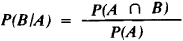which, as can be proved, corresponds completely with the properties of frequencies. Events A1, A2, …, Ar are said to be independent if the conditional probability of each of them, under the condition that some of the remaining events have occurred, is equal to its “absolute” probability.

THEOREM OF MULTIPLICATION OF PROBABILITIES. The probability of the intersection of events A1, A2, …, Ar is equal to the probability of event A1 multiplied by the probability of event A2 under the condition that A1 has occurred, …, multiplied by the probability of Ar under the condition that A1, A2, …, Ar-1 have occurred. For independent events, the multiplication theorem reduces to the formula

(3) P(A1A2 ∩ … ∩ Ar ) = P(A1 ) × P(A2 ) × … × P(Ar )

that is, the probability of the intersection of independent events is equal to the product of the probabilities of these events. Formula (3) remains correct, if on both sides some of the events are replaced by their inverses.

EXAMPLE. Four shots are fired at a target, and the hit probability is 0.2 for each shot. The target hits by different shots are assumed to be independent events. What is the probability of hitting the target three times?

Each outcome of the trial can be designated by a sequence of four letters [for example, (s, f, f, s) denotes that the first and fourth shots hit the target (success), and the second and third miss (failure)]. There are 2 · 2 · 2 · 2 = 16 outcomes in all. In accordance with the assumption of independence of the results of individual shots, one should use formula (3) and the remarks about it to determine the probabilities of these outcomes. Thus, the probability of the outcome (s, f, f, f) is set equal to 0.2 · 0.8 · 0.8 · 0.8 = 0.1024; here, 0.8 = 1 - 0.2 is the probability of a miss for a single shot. For the event “three shots hit the target,” the outcomes (s, s, s, f), (s, s, f, s), (s, f, s, s), and (f, s, s, s) are favorable and the probability of each is the same:

0.2 · 0.2 · 0.2 · 0.8 = · · · = 0.8 · 0.2 · 0.2 · 0.2 = 0.0064

Consequently, the desired probability is 4 · 0.0064 = 0.0256.

Generalizing the discussion of the given example, it is possible to derive one of the fundamental formulas of probability theory: if events A1, A2, …, An are independent and each has a probability p, then the probability of exactly m such events occurring is

Pn(m) = Cnm(1- p)n - m

Here, Cmn denotes the number of combinations of n elements taken m at a time. For large n, the calculation using formula (4) becomes difficult. In the preceding example, let the number of shots equal 100; the problem then becomes one of finding the probability x that the number of hits lies in the range from 8 to 32. The use of formula (4) and the addition theorem gives an accurate, but not a practically useful, expression for the desired probability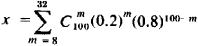The approximate value of the probability x can be found by the Laplace theorem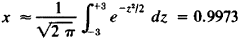with the error not exceeding 0.0009. The obtained result demonstrates that the event 8 ≤ m ≤ 32 is practically certain. This is the simplest, but a typical, example of the use of the limit theorems of probability theory.

Another fundamental formula of elementary probability theory is the so-called total probability formula: if events A1, A2, …, Ar are disjoint in pairs and their union is a certain event, then the probability of any event B is the sum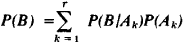The theorem of multiplication of probabilities turns out to be particularly useful in the consideration of compound trials. Let us say that trial T consists of trials T1, T2, …, Tn-1, Tn, if each outcome of trial T is the intersection of certain outcomes Ai, Bi, … , xk, Yl of the corresponding trials T1, T2, …, Tn-1, Tn. From one or another consideration, the following probabilities are often known:

(5) P(A1), P(Bj/Ai), … , P(Yi/AiBj ∩ … ∩ Xk)

According to the probabilities of (5), probabilities P(E) for all the outcomes of E of the compound trial and, in addition, the probabilities of all events connected with this trial can be determined using the multiplication theorem (just as was done in the example above). Two types of compound trials are the most significant from a practical point of view: (a) the component trials are independent, that is, the probabilities (5) are equal to the unconditional probabilities P(Ai), P(Bj), …, P(Yl); and (b) the results of only the directly preceding trial have any effect on the probabilities of the outcomes of any trial—that is, the probabilities (5) are equal, respectively, to P(Ai), P(Bj/Ai), … , P(Yl/Xk). In this case, it is said that the trials are connected in a Markov chain. The probabilities of all the events connected with the compound trial are completely determined here by the initial probabilities P(Ai) and the transition probabilities P(Bj/Ai), … , P(Yl/Xk).

RANDOM VARIABLES. If each outcome Er of a trial T is set in correspondence with a number xr, then it is said that a random variable X is assigned. The numbers x1, x2, …, xs can be equal; the set of different values of xr (r = 1, 2, …, s) is called the set of possible values of a random variable. The collection of possible values of a random variable and the probabilities corresponding to them is called the probability distribution of the random variable. Thus, in the example of throwing two dice, each outcome of the trial (i, j) is connected with a random variable X = i + j, the sum of the pips on both dice. The possible values are 2, 3, 4, …, 11, 12; the corresponding probabilities are 1/36, 2/36, 3/36, …, 2/36, 1/36.

In the simultaneous study of several random variables, one introduces the concept of their joint distribution, which is specified by the designation of the possible values of each of them and the probabilities of the intersection of events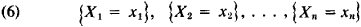where xk is any of the possible values of variable Xk. Random variables are called independent, if, for any choice of xk, the events of (6) are independent. Using a joint distribution of random variables, one can calculate the probability of any event specified by these variables; for example, of event a < X1 + X2 + · · · + Xn < b and so forth.

Often, instead of the complete specification of a probability distribution of a random variable, it is preferable to use a small number of numerical characteristics. The most frequently used are the mathematical expectation and the dispersion.

In addition to mathematical expectations and dispersions of these variables, a joint distribution of several random variables is characterized by correlation coefficients and so forth. The meaning of the listed characteristics is to a large extent explained by the limit theorems (see the section below).

A scheme of trials with a finite number of outcomes is insufficient even for the simplest applications of probability theory. Thus, in the investigation of a random spread of points around the center of a projectile’s target, in the investigation of the random errors that arise in the measurement of some quantity, and the like, it is already impossible to confine ourselves to trials with a finite number of outcomes. In addition to this, in some cases the outcome of a trial can be expressed by a number or system of numbers, while in others, the outcome of a trial can be a function (for example, the notation of the variation of pressure at a given point in the atmosphere over a given time interval), systems of functions, and so forth. It should be noted that many of the definitions and theorems given above are also applicable with essentially slight changes to more general circumstances, although the methods of specifying probability distributions vary.

The most serious change is undergone by the determination of the probability that in the elementary case is given by formula (2). More general schemes are concerned with events that are the union of an infinite number of outcomes (or, so to speak, elementary events), the probability of each of which can be equal to 0. In accordance with this, the property, expressed by the addition theorem, is not derived from the definition of probability but is included in it.

The most prevalent contemporary logical scheme of constructing the principles of probability theory was developed in 1933 by the Soviet mathematician A. N. Kolmogorov. The basic features of this scheme are the following. In the investigation of any real problem by the methods of probability theory, one first distinguishes the set U of elements w, called elementary events. Every event is completely described by the set of elementary events favorable to it and consequently is considered as a certain set of elementary events. Specific numbers P(A), called their probabilities, are connected with some of the events of A; the probabilities satisfy the following conditions:

(1) 0≤P(A)≤1

(2) P(∪) = 1

(3) If events A1, …, An are pairwise disjoint and A is their sum, then P(A) = P(A1) + P(A2) + · · · + P(An).

To create a fully valid mathematical theory, condition (3) must also be fulfilled for infinite sequences of pairwise disjoint events. The properties of nonnegativity and additivity are the basic properties of the measure of the sets. Probability theory can therefore be considered, from a formal point of view, as part of measure theory. The basic concepts of probability theory are placed in a new light with such an approach. Random variables are transformed into measurable functions, their mathematical expectations into abstract Lebesgue integrals, and so forth. However, the basic problems of probability theory and measure theory are different. The basic concept, unique for probability theory, is the concept of independence of events, trials, and random variables. In addition to this, probability theory investigates in detail such objects as conditional distributions, conditional mathematical expectations, and so forth.

Limit theorems. In the formal presentation of probability theory, limit theorems appear in the form of a superstructure over its elementary sections, in which all problems have a finite, purely arithmetic character. However, the cognitive value of probability theory is revealed only by the limit theorems. Thus, the Bernouilli theorem proves that in independent trials, the frequency of appearance of any event, as a rule, deviates little from its probability, and the Laplace theorem indicates the probabilities of one or another deviation. Analogously, the meaning of such characteristics of a random variable as its mathematical expectation and dispersion is explained by the law of large numbers and the central limit theorem. Let

(7) X1, X2, …, Xn, ...

be independent random variables that have one and the same probability distribution with EXK = a, DXK = σ2 and Yn be the arithmetic mean of the first n variables of sequence (7):

Yn = (X1 + X2 + X 2 + · · · + Xn)/n

In accordance with the law of large numbers, for any ε > 0, the probability of the inequality | Yn - a | ≤ ε has the limit 1 as n → ∞, and thus Yn, as a rule, differs little from a. The central limit theorem makes this result precise by demonstrating that the deviations of Yn from a are approximately subordinate to a normal distribution with mean zero and dispersion 82/n. Thus, to determine the probabilities of one or another deviation of Yn from a for large n, there is no need to know all the details about the distribution of the variables Xn; it is sufficient to know only their dispersion.

In the 1920’s it was discovered that even in the scheme of a sequence of identically distributed and independent random variables, limiting distributions that differ from the normal can arise in a completely natural manner. Thus, for example, if X1 is the time until the first reversion of some randomly varying system to the original state, and X2 is the time between the first and second reversions, and so on, then under very general conditions the distribution of the sum X1 + · · · + Xn (that is, of the time until the Aith reversion), after multiplication by n-1/α(α is a constant less than 1), converges to some limiting distribution. Thus, the time until the nth reversion increases, roughly speaking, as n1/α, that is, more rapidly than n (in the case of applicability of the law of large numbers, it is of the order of n).

The mechanism of the emergence of the majority of limiting regularities can be understood ultimately only in connection with the theory of random processes.

Random processes. In a number of physical and chemical investigations of recent decades, the need has arisen to consider, in addition to one-dimensional and multidimensional random variables, random processes—that is, processes for which the probability of one or another of their courses is defined. An example of a random process is the coordinate of a particle executing Brownian motion. In probability theory, a random process is usually considered as a one-parameter family of random variables X(t). In an overwhelming number of applications, the parameter t represents time, but this parameter can be, for example, a point in space, and then we usually speak of a random function. In the case when the parameter t runs through the integer-valued numbers, the random function is called a random sequence. Just as a random variable is characterized by a distribution law, a random process can be characterized by a set of joint distribution laws for X(t1), X(t2), …, X(tn) for all possible moments of t1, t2, …, tn for any n > 0. At the present time, the most interesting concrete results of the theory of random processes occur in two special areas.

Historically, Markov processes were the first to be investigated. The random process X(t) is called a Markov process if for any two moments of time t0 and t1 (t0 < t1), the conditional probability distribution of X (tx) under the condition that all the values of X(t1) are specified for tt0 depends only on X (t0) (by virtue of this, Markov random processes are sometimes called processes without consequences). Markov processes are a natural generalization of the deterministic processes considered in classical physics. In deterministic processes, the state of a system at the moment t0 uniquely determines the course of the process in the future. In Markov processes, the system’s state at the moment of time t0 determines uniquely the probability distribution of the course of the process for t > t0, and no information concerning the course of the process until the time t0 changes this distribution.

The second widely investigated area of the theory of random processes is the theory of stationary random processes. The stationariness of a process—that is, the invariability in time of its probabilistic regularities—imposes a strong restriction on the process and permits the derivation of a number of important consequences from this only assumption (for example, the possibility of a so-called spectral decomposition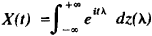where z(λ) is a random function with independent increments). At the same time, the scheme of stationary processes describes many physical phenomena to a good approximation.

The theory of random processes is closely connected with the classical problems of the limit theorems for sums of random variables. Those distribution laws that emerge in the investigation of sums of random variables as limit laws are, in the theory of random processes, exact distribution laws of corresponding characteristics. This fact permits the proof of many limit theorems using the corresponding random processes.

History. Probability theory arose in the middle of the 17th century. The first work in probability theory was done by the French scientists B. Pascal and P. Fermât and the Dutch scientist C. Huygens; it dealt with the calculation of various probabilities in games of chance. Great success in probability theory was achieved by the Swiss mathematician Jakob Bernoulli, who established the law of large numbers for a scheme of independent trials with two outcomes (published in 1713).

The following (second) period in the history of probability theory (18th century and beginning of the 19th century) is associated with the names of A. Moivre (England), P. Laplace (France), K. Gauss (Germany), and S. Poisson (France). This was a period when probability theory already found a number of actual applications in natural science and technology (chiefly in the theory of errors of observation developed in connection with the requirements of geodesy and astronomy and in the theory of shooting). The proofs of the first limit theorems, which are now called the Laplace (1812) and Poisson (1837) theorems, were done in this period. A. Legendre (France, 1806) and K. Gauss (1808) developed the method of least squares.

The third period in the history of probability theory (second half of the 19th century) is associated primarily with the names of the Russian mathematicians P. L. Chebyshev, A. M. Liapunov, and A. A. Markov (the elder). Probability theory developed in Russia before this period. (In the 18th century a number of works on probability theory were written by L. Euler, N. Bernoulli, and D. Bernoulli working in Russia; in the second period of the development of probability theory, one should note the works of M. V. Ostrogradskii on problems connected with mathematical statistics and V. Ia. Buniakovskii on applications of probability theory to insurance, statistics, and demography.) Beginning in the second half of the 19th century, Russia was the leader in investigations in probability theory. Chebyshev and his students Liapunov and Markov stated and solved a number of general problems in probability theory, generalizing the theorems of Bernoulli and Laplace. Chebyshev very simply proved (1867) the law of large numbers under very general assumptions. He first formulated (1887) the central limit theorem for sums of independent random variables and indicated one of the methods of proving it. Using another method, Liapunov obtained (1901) a solution that was close to the definitive solution of this problem. Markov first considered (1907) one case of dependent trials, which was subsequently called the Markov chain.

In Western Europe in the second half of the 19th century, great development occurred in mathematical statistics (in Belgium, L. A. J. Quetelet, and in England, F. Galton) and statistical physics (in Austria, L. Boltzmann), which, together with the fundamental theoretical works of Chebyshev, Liapunov, and Markov, created the basis for the substantial broadening of problems in probability theory in the fourth (modern) period of its development. This period in the history of probability theory is characterized by an extremely broad range of applications and by the creation of several systems of completely strict mathematical bases of probability theory and powerful new methods (except classical analysis) sometimes requiring the use of the methods of set theory, the theory of functions of a real variable, and functional analysis. In this period, with a great intensification of work in probability theory abroad (in France, E. Borel, P. Lévy, M. Fréchet; in Germany, R. Mises; in the USA, N. Wiener, W. Feller, J. Doob; and in Sweden, H. Cramer), Soviet science continues to occupy a significant, and in a number of fields the leading, position. In the Soviet Union, a new period of development of probability theory has been begun by S. N. Bernshtein, who has considerably generalized the classical limit theorems of Chebyshev, Liapunov, and Markov and has, for the first time in Russia, conducted intensive work on applications of probability theory to natural science. In Moscow, A. Ia. Khinchin and A. N. Kolmogorov began with the application of the methods of the theory of functions of a real variable to the problems of probability theory. Later (in the 1930’s) they, together with E. E. Slutskii, laid the foundations of the theory of random processes. V. I. Romanovskii (Tashkent) and N. V. Smirnov (Moscow) raised work in the applications of probability theory to mathematical statistics to a high level. Besides the large Moscow group of specialists in probability theory, the problems of probability theory in the USSR are studied in Leningrad (headed by lu. V. Linnik) and in Kiev at the present time.

### REFERENCES

#### Founders and classics in probability theory

Bernoulli, J. Ars conjectandi, opus posthumum. Basel, 1713. (Russian translation, St. Petersburg, 1913.)
Laplace [P. S.] Théorie analytique des probabilités, 3rd ed. Paris, 1886. (Oeuvres complètes de Laplace, vol. 7, books 1-2.)
Chebyshev, P. L. Poln. sobr. soch, vols. 2-3. Moscow-Leningrad, 1947-48.
Liapunov, A. Nouvelle forme du théorème sur la limite de probabilité. St. Petersburg, 1901. (Zap. AN po fiziko-matematiche-skomu otdeleniiu, 8 seriia, vol. 12, no. 5.)
Markov, A. A. “Issledovanie zamechatel’nogo sluchaia zavisimykh ispytanii.” Izv. AN, 6 seriia, vol. 1, no. 3, 1907.

#### Popular and textbook literature

Gnedenko, B. V., and A. Ia. Khinchin. Elementarnoe vvedenie v teoriiu veroiatnostei, 3rd ed. Moscow-Leningrad, 1952.
Gnedenko, B. V. Kurs teorii veroiatnostei, 4th ed. Moscow, 1965.
Markov, A. A. Ischislenie veroiatnostei, 4th ed. Moscow, 1924.
Bernshtein, S. N. Teoriia veroiatnostei, 4th ed. Moscow-Leningrad, 1946.
Feller, W. Vvedenie v teoriiu veroiatnostei i ee prilozhenie (Diskretnye raspredeleniia), 2nd ed., vols. 1-2. Moscow, 1967. (Translated from English.)

#### Surveys and monographs

Gnedenko, B. V., and A. N. Kolmogorov. “Teoriia veroiatnostei.” In Matematika v SSSR za tridtsat’ let, 1917-1947. Moscow-Leningrad, 1948. (Collection of articles.)
Kolmogorov, A. N. “Teoriia veroitanostei.” In Matematika v SSSR za sorok let, 1917-57, vol. 1. Moscow, 1959. (Collection of articles.)
Kolmogorov, A. N. Osnovnye poniatiia teorii veroiatnostei. Moscow-Leningrad, 1936. (Translated from German.)
Kolmogorov, A. N. “Ob analiticheskikh metodakh v teorii veroiatnostei.” Uspekhi matematicheskikh nauk, 1938, issue 5, pp. 5-41.
Khinchin, A. Ia. Asimptoticheskie zakony teorii veroiatnostei. Moscow-Leningrad, 1936. (Translated from German.)
Gnedenko, B. V., and A. N. Kolmogorov. Predel’nye raspredeleniia dlia summ nezavisimykh sluchainykh velichin. Moscow-Leningrad, 1949.
Doob, J. L. Veroiatnostnye protsessy. Moscow, 1956. (Translated from English.)
Chandrasekar, S. Stokhasticheskie problemy v fizike i astronomii. Moscow, 1947. (Translated from English.)
Prokhorov, Iu. V., and Iu. A. Rozanov. Teoriia veroiatnostei. Moscow, 1967.

IU. V. PROKHOROV and B. A. SEVAST’IANOV

References in periodicals archive ?
In the late 1800's and early 1900's, statistical methods and probability theory developed significantly.
"In our system, a probability is a theoretical construct, on the epistemological level, which we assign in order to represent a state of knowledge, or that we calculate from other probabilities according to the rules of probability theory. A frequency is a property of the real world, on the ontological level, that we measure or estimate."
Also in 1956, appeared a treatise on Probability Theory and Applications, co-authored by Onicescu, Mihoc and Ionescu Tulcea, a work distinguished with the State Prize in 1962.
Chapter 3 introduces stochastic processes in discrete time based on the probability theory framework described in the previous chapter.
Risk assessment is currently based on the implicit premise that probability theory provides the necessary and sufficient tools for dealing with risk and variability.
However, Keynes' development of an objective account of logical probability in the Treatise on Probability (1921) can be read as departing from Moore by showing how probability theory could be used to mitigate uncertainties.
A justified functional U in theory T has been found, which for each function u in the theory measures the amount of uncertainty associated with u (an example of functional U is the well-known Shannon entropy in probability theory).
Who would have thought it, but Nugent presents a look at probability theory's relevance to assessment and diagnosis, coming to the same negative conclusion that others in the book do; Nugent's specific words connect diagnostic inference to roulette and black jack.
This book is the only reference of its kind that uses probability theory to quantify the management appraisal process, says the publisher.
Probability theory's value as a mathematical tool in decision-making, and especially in games of chance, dates back at least to the First Dynasty in Egypt (c.3500 B.C.).
The work of Amos Tversky and Daniel Kahneman showed that the same heuristics that help people make daily decisions under uncertainty also lead to "severe and systematic errors" that contradict established probability theory (1974, p.
Some simple algebra and probability theory is involved; the step-by-step details and highlighted problem-solving charts make Business Case Analysis a superb guideline for readers of all backgrounds to quickly get acquainted with the theories.

Site: Follow: Share:
Open / Close